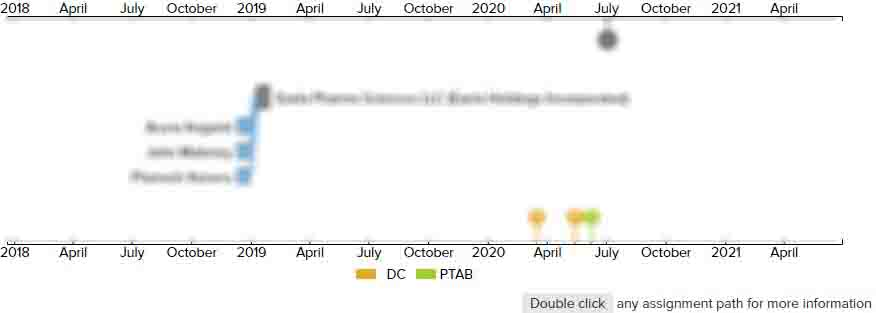# METHOD FOR SUB-PIXEL VALUE INTERPOLATION

• US 20080069203A1
• Filed: 08/15/2007
• Published: 03/20/2008
• Est. Priority Date: 09/17/2001
• Status: Active Grant
##### First Claim
Patent Images

1. A method for sub-pixel value interpolation to determine values for sub-pixels situated within a rectangular bounded region defined by four corner pixels with no intermediate pixels between the corners, the pixels and sub-pixels being arranged in rows and columns, the pixel and sub-pixel locations being representable mathematically within the rectangular bounded region using the co-ordinate notation K/2N, L/2N, K and L being positive integers having respective values between zero and 2N, N being a positive integer greater than one and representing a particular degree of sub-pixel value interpolation, the method comprising:

• interpolating a sub-pixel value for a sub-pixel having co-ordinates with odd values of both K and L, according to a predetermined choice of a weighted average of the value of a nearest-neighbouring pixel and the value of the sub-pixel situated at co-ordinates ½

, ½

, and a weighted average of the values of a pair of diagonally-opposed sub-pixels having co-ordinates with even values of both K and L, including zero, situated within a quadrant of the rectangular bounded region defined by corner pixels having co-ordinates ½

, ½ and

the nearest neighbouring pixel;

interpolating sub-pixel values for sub-pixels having co-ordinates with K equal to an even value and L equal zero and sub-pixels having co-ordinates with K equal to zero and L equal to an even value, used in the interpolation of the sub-pixels having co-ordinates with odd values of both K and L, using weighted sums of the values of pixels located in rows and columns respectively; and

interpolating sub-pixel values for sub-pixels having co-ordinates with even values of both K and L, used in the interpolation of sub-pixel values for the sub-pixels having co-ordinates with odd values of both K and L, using a predetermined choice of either a weighted sum of the values of sub-pixels having co-ordinates with K equal to an even value and L equal to zero and the values of sub-pixels having corresponding co-ordinates in immediately adjacent rectangular bounded regions, or a weighted sum of the values of sub-pixels having co-ordinates with K equal to zero and L equal to an even value and the values of sub-pixels having corresponding co-ordinates in immediately adjacent bounded rectangular regions.

• ##### 2 Assignments
Timeline View
Assignment View×
×